# STOICHIOMETRY What is stoichiometry l Stoichiometry is the

• Slides: 21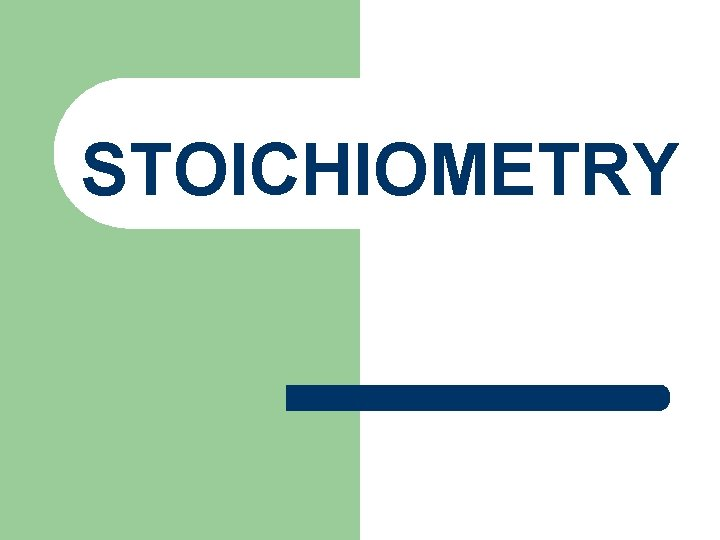STOICHIOMETRY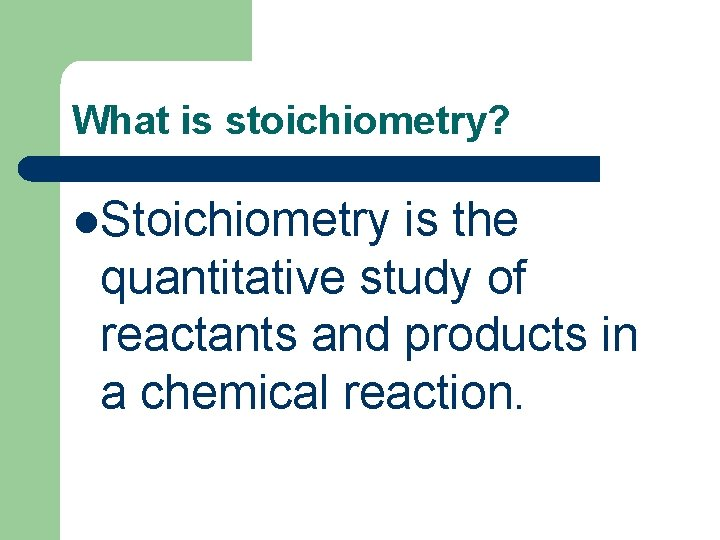What is stoichiometry? l. Stoichiometry is the quantitative study of reactants and products in a chemical reaction.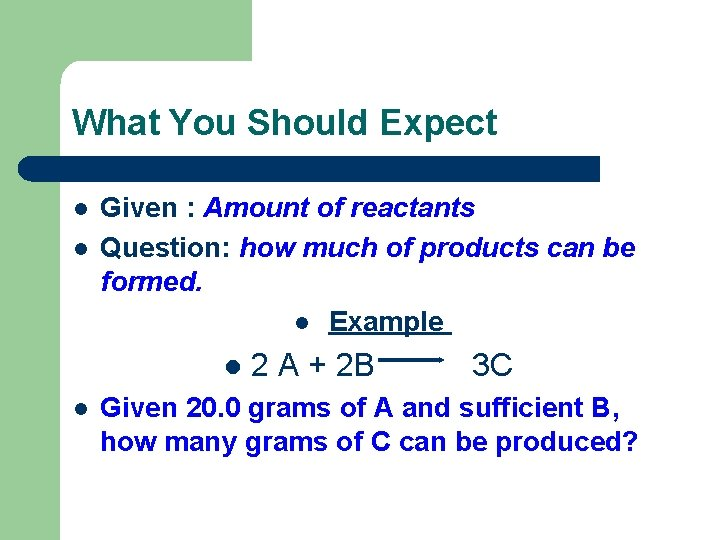What You Should Expect l l Given : Amount of reactants Question: how much of products can be formed. l Example l l 2 A + 2 B 3 C Given 20. 0 grams of A and sufficient B, how many grams of C can be produced?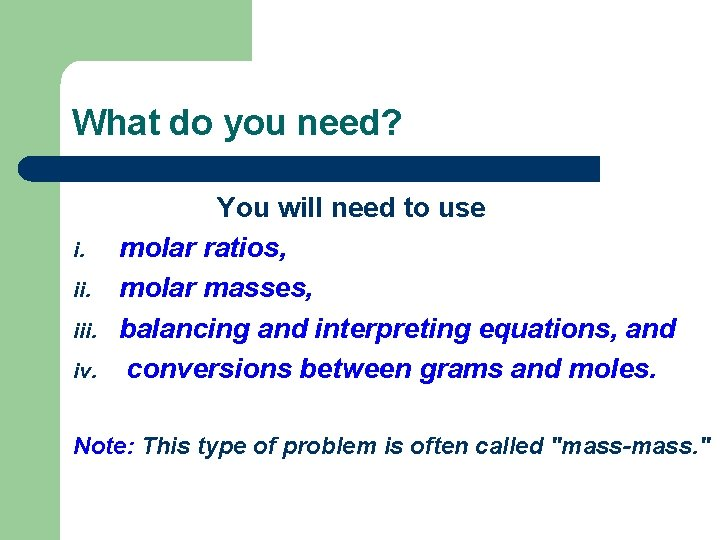What do you need? i. iii. iv. You will need to use molar ratios, molar masses, balancing and interpreting equations, and conversions between grams and moles. Note: This type of problem is often called "mass-mass. "Steps Involved in Solving Mass-Mass Stoichiometry Problems l l Balance the chemical equation correctly Using the molar mass of the given substance, convert the mass given to moles. Construct a molar proportion (two molar ratios set equal to each other) Using the molar mass of the unknown substance, convert the moles just calculated to mass.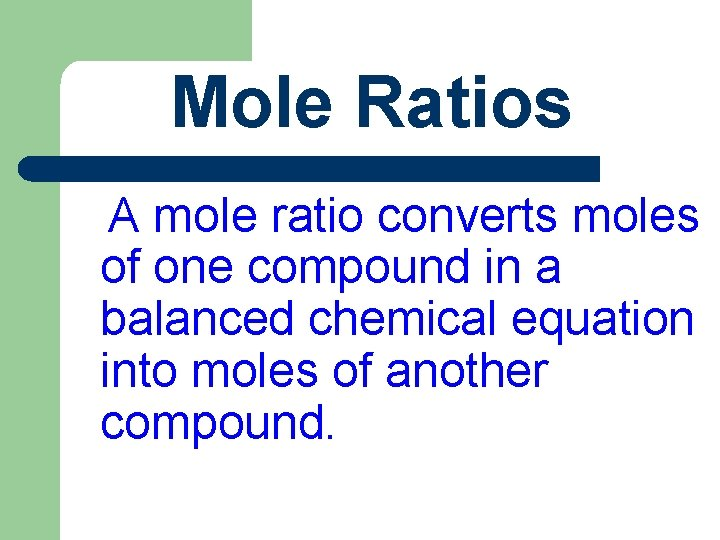Mole Ratios A mole ratio converts moles of one compound in a balanced chemical equation into moles of another compound.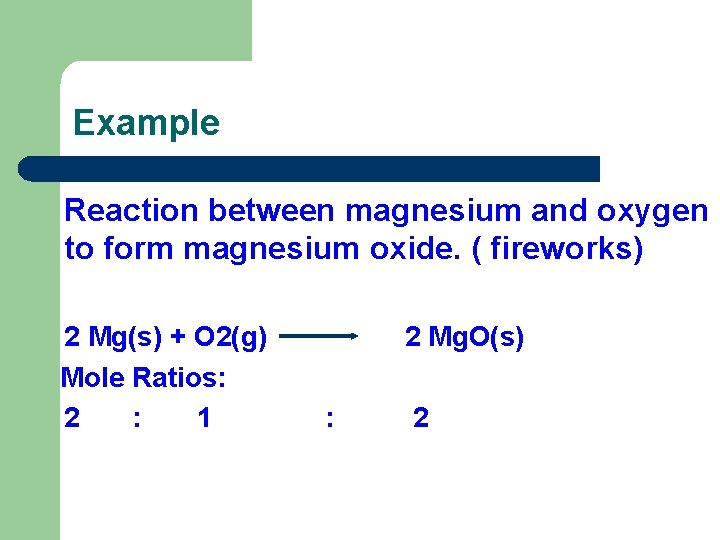Example Reaction between magnesium and oxygen to form magnesium oxide. ( fireworks) 2 Mg(s) + O 2(g) Mole Ratios: 2 : 1 2 Mg. O(s) : 2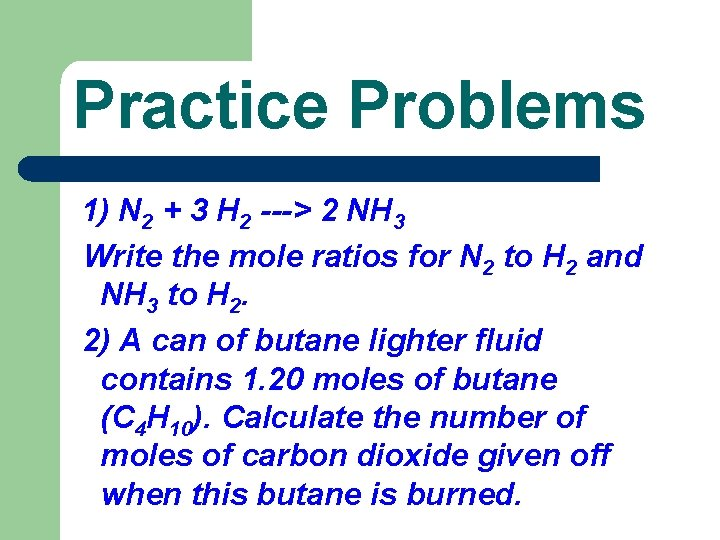Practice Problems 1) N 2 + 3 H 2 ---> 2 NH 3 Write the mole ratios for N 2 to H 2 and NH 3 to H 2. 2) A can of butane lighter fluid contains 1. 20 moles of butane (C 4 H 10). Calculate the number of moles of carbon dioxide given off when this butane is burned.Mole-Mole Problems Using the practice question 2) above: Equation of reaction 2 C 4 H 10 + 13 O 2 8 CO 2 + 10 H 2 O Mole ratio C 4 H 10 CO 2 1 : 4 [ bases] 1. 2 : X [ problem] By cross-multiplication, X = 4. 8 mols of CO 2 given off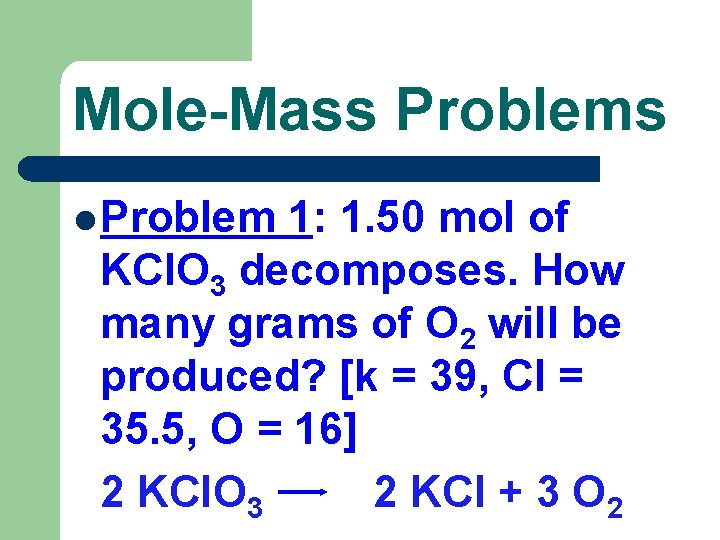Mole-Mass Problems l Problem 1: 1. 50 mol of KCl. O 3 decomposes. How many grams of O 2 will be produced? [k = 39, Cl = 35. 5, O = 16] 2 KCl. O 3 2 KCl + 3 O 2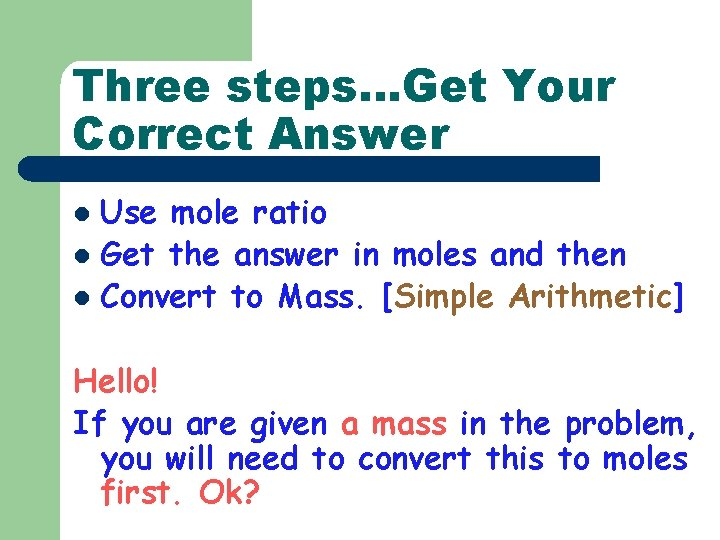Three steps…Get Your Correct Answer Use mole ratio l Get the answer in moles and then l Convert to Mass. [Simple Arithmetic] l Hello! If you are given a mass in the problem, you will need to convert this to moles first. Ok?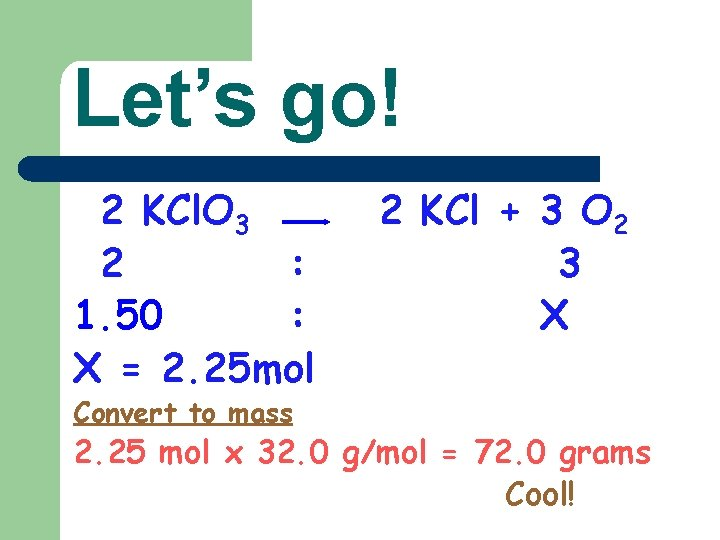Let’s go! 2 KCl. O 3 2 : 1. 50 : X = 2. 25 mol Convert to mass 2 KCl + 3 O 2 3 X 2. 25 mol x 32. 0 g/mol = 72. 0 grams Cool!Try This: We want to produce 2. 75 mol of KCl. How many grams of KCl. O 3 would be required? Soln l KCl. O 3 : KCl 2 : 2 X : 2. 75 X = 2. 75 mol In mass: 2. 75 mol X 122. 55 g/mol = 337 grams zooo zimple!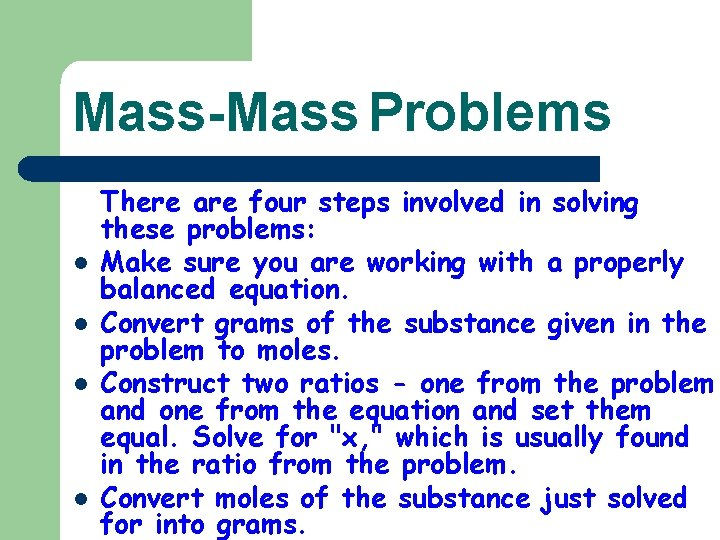Mass-Mass Problems l l There are four steps involved in solving these problems: Make sure you are working with a properly balanced equation. Convert grams of the substance given in the problem to moles. Construct two ratios - one from the problem and one from the equation and set them equal. Solve for "x, " which is usually found in the ratio from the problem. Convert moles of the substance just solved for into grams.Mass-Volume Problems Just follow mass problem to the penultimate level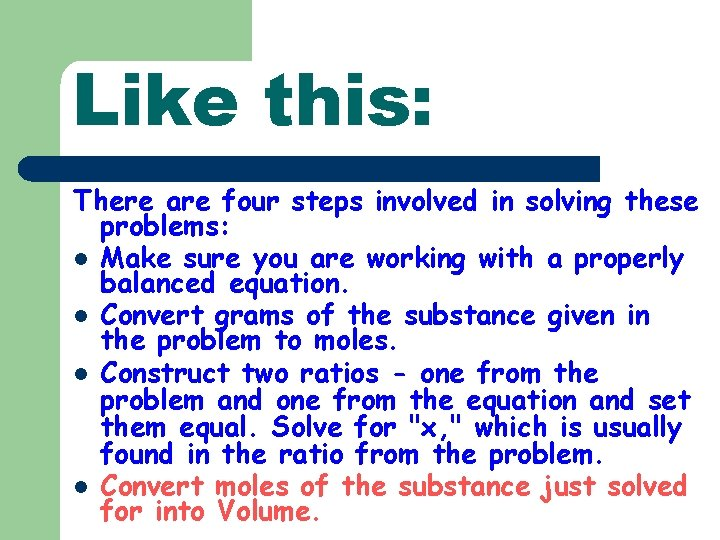Like this: There are four steps involved in solving these problems: l Make sure you are working with a properly balanced equation. l Convert grams of the substance given in the problem to moles. l Construct two ratios - one from the problem and one from the equation and set them equal. Solve for "x, " which is usually found in the ratio from the problem. l Convert moles of the substance just solved for into Volume.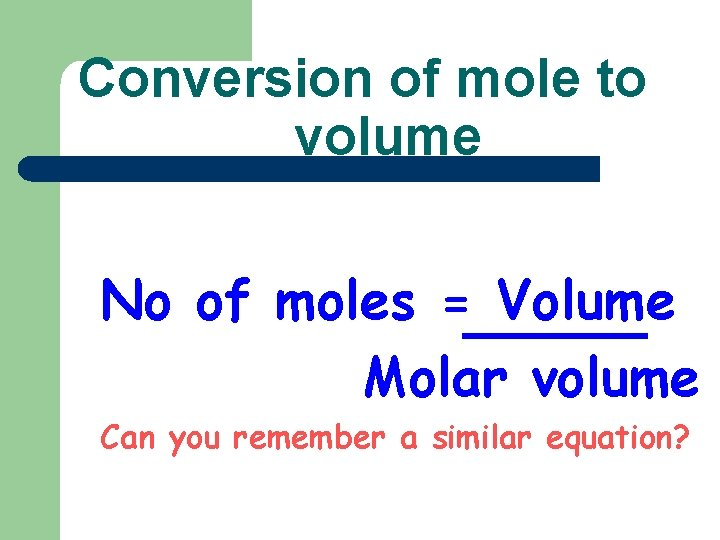Conversion of mole to volume No of moles = Volume Molar volume Can you remember a similar equation?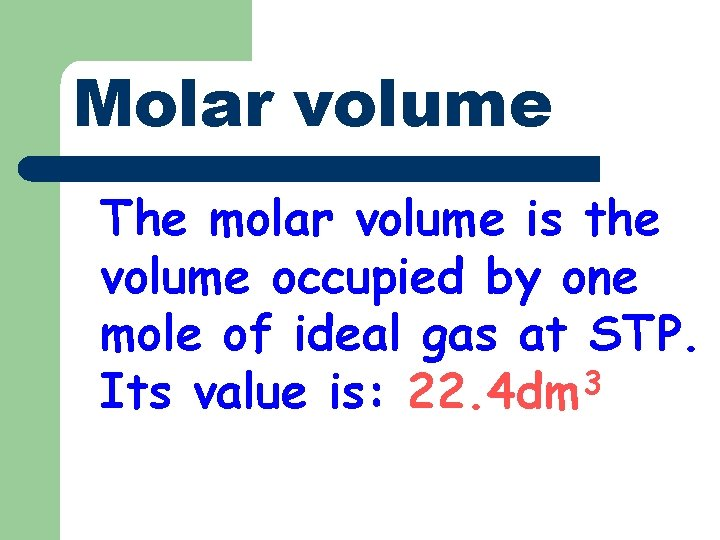Molar volume The molar volume is the volume occupied by one mole of ideal gas at STP. Its value is: 22. 4 dm 3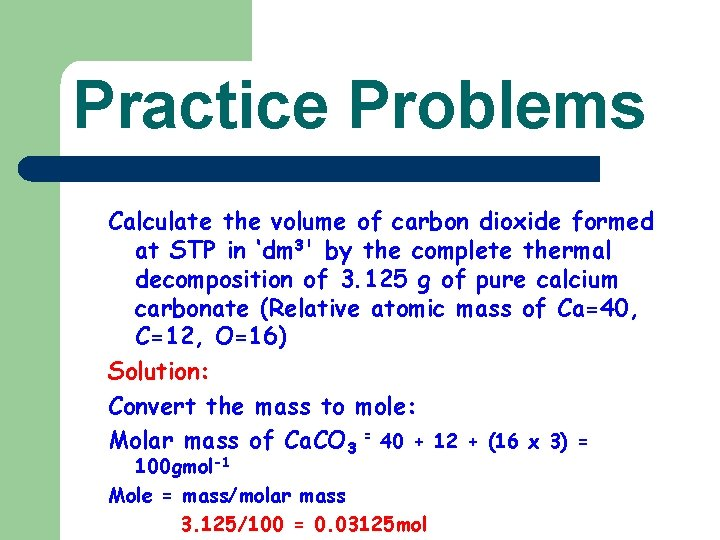Practice Problems Calculate the volume of carbon dioxide formed at STP in ‘dm 3' by the complete thermal decomposition of 3. 125 g of pure calcium carbonate (Relative atomic mass of Ca=40, C=12, O=16) Solution: Convert the mass to mole: Molar mass of Ca. CO 3 = 40 + 12 + (16 x 3) = 100 gmol-1 Mole = mass/molar mass 3. 125/100 = 0. 03125 mol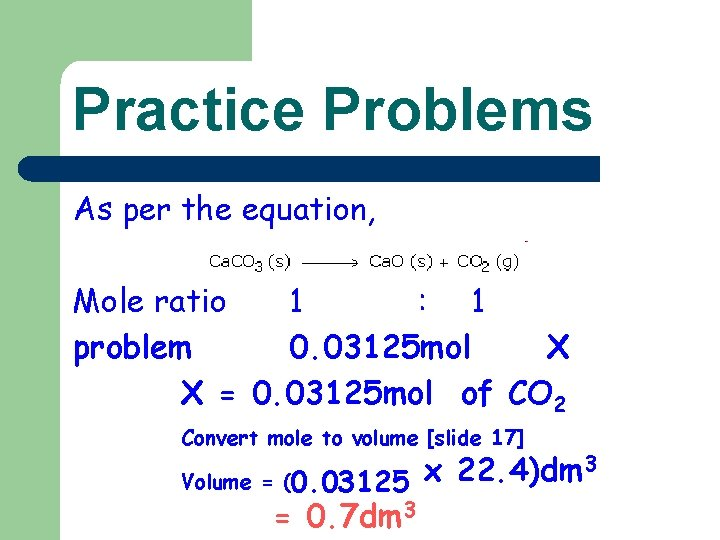Practice Problems As per the equation, Mole ratio 1 : 1 problem 0. 03125 mol X X = 0. 03125 mol of CO 2 Convert mole to volume [slide 17] 3 x 22. 4)dm Volume = (0. 03125 = 0. 7 dm 3This powerpoint was kindly donated to www. worldofteaching. com http: //www. worldofteaching. com is home to over a thousand powerpoints submitted by teachers. This is a completely free site and requires no registration. Please visit and I hope it will help in your teaching.